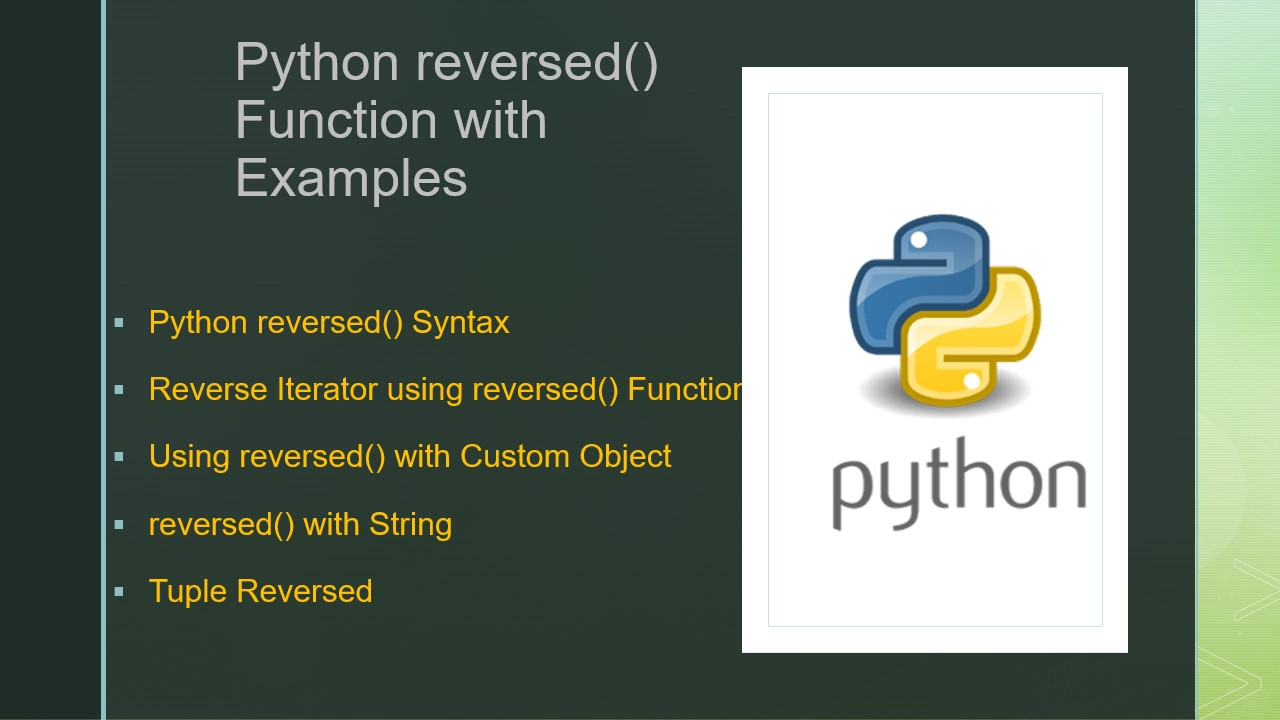# Python reversed() Function with Examples

The reversed() is a built-in function in Python that is used to reverse any iterable objects. This function takes the iterable object as an argument, reverse the elements, and returns the list_reverseiterator. You need to convert this to your respective iterable object.

## 1. Python reversed() Syntax

Following is the syntax of the reversed() function.

``````
#Syntax
reversed(iterable)
``````

### 1.1 reversed() Parameters

• iterable/sequence – Takes iterable objects like list, tuple, dictionary e.t.c

### 1.2 Return Type

• This function returns the type of `list_reverseiterator` with the reversed iterable object.

## 2. Reverse Iterator using reversed() Function

The reversed() is a built-in function in python that will reverse any iterable like a list, tuple, dictionary etc, and returns a `list_reverseiterator`, this needs to convert back to the iterable object you want. For example, if you want the result in the list, use the `list(list_reverseiterator)`.

The `type(reversed(countries))` returns the `<class 'list_reverseiterator'>`.

The below examples take the list of strings and reverse the elements inthe list by using reversed() function.

``````
# Consider list of countries
countries=["India","China","Russia","China"]
print("Actual List: ",countries)

# Using reversed()
result = reversed(countries)
print("Return Type of reversed(): "+str(type(result)))
print("Reversed List: ",list(result))
``````

This example yields the below output.

## 3. Using reversed() with Custom Object

Create a custom object by using the reversed() function; let’s reverse the elements in the object.

``````
# Custom class
class numbers:
number = [10,9,8,7,6,5]

# Custom reversed function
def __reversed__(self):
return reversed(self.number)

# Main Function
if __name__ == '__main__':
obj = numbers()
print(list(reversed(obj)))

# Output:
# [5, 6, 7, 8, 9, 10]
``````

## 4. reversed() with String

Sometimes you would be required to get the string reversed; you can easily achieve this by using reversed() however, notice that this function returns the reversed character of the string as an iterable and when you use list() to convert you will get the list of character in reverse order.

``````
# reverse String
mystring = "PYTHON"
result = reversed(mystring)
print("Original: ", mystring)
print("Reversed: ",list(result))

# Output:
Original: PYTHON
Reversed: ['N', 'O', 'H', 'T', 'Y', 'P']
``````

## 5. Tuple Reversed

Let’s perform the tuple reversed. In the below example, tuple() function converts the reversed object back to a tuple.

``````
# tuple reversed
mytuple = (1,2,3,'a','e')
result = reversed(mytuple)
print("Original: ", mytuple)
print("Reversed: ",tuple(result))

# Output:
# Original:  (1, 2, 3, 'a', 'e')
# Reversed:  ('e', 'a', 3, 2, 1)
``````

## Conclusion

In this article, you have learned the syntax of the reversed() built-in function, its parameters, and how it returned the reversed list. This function takes the iterable as an argument, reverse the elements, and returns the list_reverseiterator object. You can convert this to any iterable object, for example, to convert to a list use list().

For more functions, refer to Python Built-in Functions

### Naveen

I am a Data Engineer with 20+ years of experience in transforming data into actionable insights. Over the years, I have honed my expertise in designing, implementing, and maintaining data pipelines with frameworks like Apache Spark, PySpark, Pandas, R, Hive and Machine Learning. My journey in the field of data engineering has been a continuous learning, innovation, and a strong commitment to data integrity. I have started this SparkByExamples.com to share my experiences with the data as I come across. You can learn more about me at LinkedIn